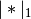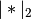# statsmodels.duration.hazard_regression.PHReg.fit_regularized¶

`PHReg.``fit_regularized`(method='coord_descent', maxiter=100, alpha=0.0, L1_wt=1.0, start_params=None, cnvrg_tol=1e-07, zero_tol=1e-08, **kwargs)[source]

Return a regularized fit to a linear regression model.

Parameters: method : Only the coordinate descent algorithm is implemented. maxiter : integer The maximum number of iteration cycles (an iteration cycle involves running coordinate descent on all variables). alpha : scalar or array-like The penalty weight. If a scalar, the same penalty weight applies to all variables in the model. If a vector, it must have the same length as params, and contains a penalty weight for each coefficient. L1_wt : scalar The fraction of the penalty given to the L1 penalty term. Must be between 0 and 1 (inclusive). If 0, the fit is a ridge fit, if 1 it is a lasso fit. start_params : array-like Starting values for params. cnvrg_tol : scalar If params changes by less than this amount (in sup-norm) in once iteration cycle, the algorithm terminates with convergence. zero_tol : scalar Any estimated coefficient smaller than this value is replaced with zero. A PHregResults object, of the same type returned by fit.

Notes

The penalty is the”elastic net” penalty, which is a convex combination of L1 and L2 penalties.

The function that is minimized is: ..math:

```-loglike/n + alpha*((1-L1_wt)*|params|_2^2/2 + L1_wt*|params|_1)
```

whereandare the L1 and L2 norms.

Post-estimation results are based on the same data used to select variables, hence may be subject to overfitting biases.